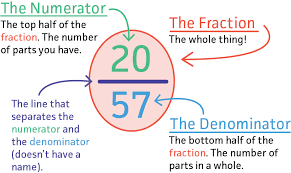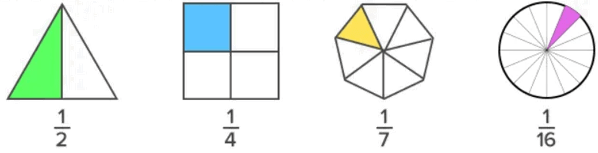#Terminology Used in Math Fractions

List of fraction vocabulary.Click the below words to see the vocabulary explanation.

#### 1) What is Fraction

A fraction is a part of a whole.

Example:#### 2) What is Numerator

It can be defined as the top number in a fraction.

Example:

20/57, 20 is the numerator

#### 3) What is Fraction Bar

The line that separates the numerator and the denominator. Also called a Vinculum.

#### 4) What is Denominator

It can be defined as the bottom number in a fraction.It is the divisor of a fraction.

Example:

20/57, 57 is the denominator, meaning there are 57 parts altogether.

#### 5) What is Common Factor

A whole number that will divide exactly into two or more given numbers without leaving a remainder.

Example:

Factor of 6 = 1, 2, 3 and 6.

Factor of 8 = 1, 2, 4 and 8.

Common factors of 6 and 8 = 1 and 2.

#### 6) What is Common Multiple

A whole number that is a shared multiple of each of set of numbers.

Example:

Multiples of 2 are 2, 4, 6, 8, 10, 12, 14, 16, 18

Multiples of 3 are 3, 6, 9, 12, 15, 18

Common multiples of 2 and 3 are 6, 12, 18

#### 7) What is Equivalent Fraction

It can be defined as fractions with different numberators and denominators that represent the same value or proportion of the whole.

Example:

1/2 + 1/2

#### 8) What is Factor

It can be defined as the number we multiply with another number to get a product.

Example:

6 = 3 × 2

Factors of 6 are 3 and 2

#### 9) What is Factor Pairs

A factor pair refers to a set of two numbers, which when multiplied result in a definite number.

Example:

2 × 5 = 10, 2 and 5 is one of the factor pair that gives us the product 10.

In other words, 10 is the product of 2 and 5

In other words, 10 is the multiple of 2 and 5, and here, we are multiplying 2 and 5 to get 10. Hence, 2 and 5 are the factors of 10.

#### 10) What is Fraction Greater Than One

A fraction where the numerator is higher than denominator.#### 11) What is Fraction Less Than One

A fraction where the numerator is lower than denominator.

#### 12) What is Frequency Table

A frequency table is a table that lists items and show the number of times the items occur.

#### 13) What is Like Denominators

Two fractions having same denominators..

#### 14) What is Like Numerators

Two fractions having same numerators.

#### 15) What is Unit Fraction

It can be defined as a fraction whose numerator is 1.

Example:#### 16) What is Unlike Denominators

Two fractions where denominators are not identical or similar.

Example:

3/4 + 1/5

#### 17) What is Unlike Numerators

Two fractions where numerators are not identical or similar.

Example:

3/4 + 1/5

#### 18) What is Complex Fraction

A fraction that contains another fraction in its numertor and/or denominator.

#### 19) What is Proper Fraction

The numerator is less than the denominator.

Example:

5/6

The value is less than 1

#### 20) What is Improper Fraction

The numerator is more than the denominator.

Example:

8/6

The value is more than 1

#### 21) What is Mixed Number

Whole number part and a fraction part.

Example:

1 2/6

The value is more than 1